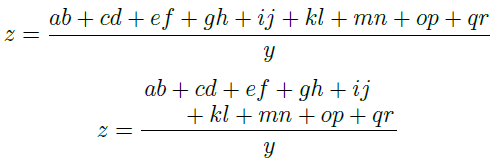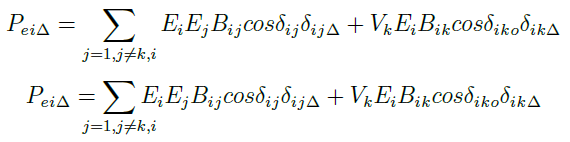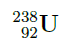Showing first {{hits.length}} results of {{hits_total}} for {{searchQueryText}}{{hits.length}} results for {{searchQueryText}}

Aucun résultat pour la recherche

Author: Robert Murrish (June 2012) mathtools is the package you likely never knew you needed. LaTeX makes typesetting equations easy, and mathtools makes those equations beautiful. mathtools is an extention of amsmath. If you include mathtools, you can use any function or macro from that package. amsmath is a broad set of tools for typesetting equations, and is widely used. mathtools extends the amsmath functions to provide additional formatting options and to clean up some of the more common problems with math typesetting. A few examples Two-line fractions A complicated fraction can extend so wide that it exceeds the column width. Splitting the numerator or/and denominator into multiple lines is a classy way to keep it together. z=\frac{ab+cd+ef+gh+ij+kl+mn+op+qr}{y} z=\frac{\splitfrac{ab+cd+ef+gh+ij}{+kl+mn+op+qr}}{y}Long summation parameters Without the mathtools function \mathclap, there are extra spaces created on either side of the summation symbol, because of the indices below. A simple mathtools command condenses the result. P_e_i_\Delta = \sum_{j=1, j\not=k,i}E_iE_jB_i_j cos\delta_i_j\delta_i_j_\Delta + V_kE_iB_i_k cos\delta_i_k_o \delta_i_k_\Delta P_e_i_\Delta = \sum_{\mathclap{j=1, j\not=k,i}}E_iE_jB_i_j cos\delta_i_j \delta_i_j_\Delta + V_kE_iB_i_k cos\delta_i_k_o \delta_i_k_\DeltaPrescripts Allows superscripts and subscripts to the left of (before) a symbol. Here's a chemistry example: \prescript{238}{92}{\mathbf{U}}These full examples are a good place to start exploring mathtools, but there are many more tools available in this package. Below is a full listing of all the macros contained in the package (as of the date this article was written). For an up-to-date list, and specific details about how to use each one, see the documentation for the mathtools package. Function Name Description mathclap This function typesets its argument without taking any horizontal space, protruding symmetrically on both sides. As shown above, this function can be used to remove some stray whitespace that can occur in subscripts, exponents, etc. mathrlap mathllap Same as mathclap, but only protrudes to the right or left side, respectively. cramped Similar to mathclap, this condenses certain items. For example, it brings exponents in a little tighter, which can be useful especially with more than one level of exponents. crampedclap crampedrlap crampedllap Applies both cramped and mathclap (or mathrlap, etc.). Same effect as applying both separately, but with a performance boost in the processing. smashoperator Condenses text like a combination of all the functions above. It's simpler to use in most cases, but you lose some control and may not be so pleased with the result. In that case, use the functions above individually. adjustlimits Works on limits (\lim), to make more complex limits align vertically. SwapAboveDisplaySkip Elliminates some of the whitespace above an equation. newtagform renewtagform usetagform The "tags" are the equations numbers, and this set of functions allows you to modify them, e.g., change the parentheses, style, etc. refeq An alternative way to reference functions within the document text, without causing font mis-match problems. showonlyrefs This is a flag, not a function, but still quite useful. If set, only equations referenced in the text will receive equation numbers. showmanualtags Same as showonlyrefs, but for equations where manual tags have been set. noeqref Allows you to create an exception to the two flags above, to show an equation number or tag, even if it is not referenced in the text. xleftrightarrow xLeftarrow xRightarrow xhookleftarrow xhookrightarrow xmapsto A set of functions for drawing various arrows that will expand as needed to fit the associated text. xrightharpoondown xleftharpoondown xrightharpoonup xleftharpoonup xrightleftharpoons xleftrightharpoons A set of 'harpoons' or vertical arrows. Similar to the arrows, they will expand as needed overbrace underbrace Puts a brace over or under equation. Same as the functions built into LaTeX, but will often typeset better. You can use \LaTeXoverbrace and \LaTeXunderbrace if you need the original LaTeX functions. overbracket underbracket Goes over or under an equation like overbrace and underbrace, but with square brakets. begin{matrix} and friends There are several options for typesetting matrices. They mostly serve to center elements better than the default settings, but have some other options as well, like smallmatrix. I suggest you look at the official mathtools documentation to determine which would serve your need best, once you have a matrix defined. multlined Split a long equation into parts, similar to the split fractions in the first example above. begin{cases} and friends Tools to properly typeset piecewise equations. Again, there are many options available, rather than cover them all, I refer you to the official mathtools documentation once you know what your piecewise equation looks like. MoveEqLeft A way to produce indentation for equations if necessary. Aboxed Draws a box around an equation. ArrowBetweenLines vdotswithin shortvdotswithin MTFlushSpaceAbove MTFlushSpaceBelow These functions allow for drawing in the whitespace between equations, arrows, dots, etc. shortintertext Like the function \intertext in amsmath, this allows for inserting a bit of text in a series of equations without upsetting the alighment. This version of the function produces less whitespace. DeclarePairedDelimiter Allows for defining new delimiters, and example from the documentation is for creating a set of absolute value bars the proper height for a fraction. This function has some advanced options available. lparen rparen Equivalent to \left(, \right). Provided for compatibility. vcentcolon Draws a colon centered vertically. nuparrow ndownarrow bigtimes Provides characters that are sometimes not otherwise available. prescript Allows super- and subscripts before a character, as shown in example 3 above. begin{spreadlines} The spreadlines environment allows you to explicitly define the vertical spacing between equations. splitfrac splitdfrac Allows for split numerators or denominators in fractions, as in example 1 above. The following are direct links to some of the mathtools documentation available: mathtools packge and documentation on CTAN; article in TUGboat.